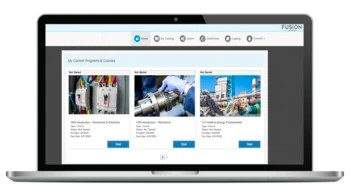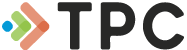• Online Training• Instructor-Led Training• Performance• Compliance• TECHNOLOGY• Industries• Resources• Contact
• ## Fundamentals# Mathematics in the Plant

Begins by introducing mathematical basics-numbers and numerals, subtraction, addition, multiplication, and division. This online course examines common fractions and decimal fractions, ratios and proportions, powers and roots. Discusses the calculator: usage, basic and special functions, internal logic, and special purpose calculators. Moves on to cover geometry, algebra, and formulas for problem solving. Concludes by explaining properties of triangles and trig and inverse trig functions. This course has no prerequisites. Mathematics in the Plant is available in online technical training and course manual formats.

Review a full training course list for Instrumentation and Control Technician

Back to Fundamentals Courses

#### Select Format

• Online Course & Test \$85.00
• Course Manual \$71.00
• Course Manual (Spanish) \$71.00

TPC Training is authorized by IACET to offer 1.0 CEUs for Mathematics in the Plant online training.

#### Lesson 1 - Whole Numbers

##### Topics:

Numbers and numerals; Decimal system; Positive and negative numbers; Addition; Multiplication; Subtraction; Division

##### Learning Objectives:
– Describe the difference between a number and a numeral.
– Demonstrate how to add three four-digit numbers, with carrying.
– Demonstrate how to subtract two four-digit numbers, with borrowing.
– Demonstrate how to multiply a four-digit number by a two-digit number.
– Demonstrate how to divide a four-digit number by a two-digit number.

#### Lesson 2 - Common Fractions

##### Topics:

Fraction definition; Improper fractions; Mixed numbers; Reducing fractions; Adding, subtracting, multiplying, and dividing fractions

##### Learning Objectives:
– State the definition of a fraction.
– Demonstrate how to reduce a fraction to its lowest terms.
– Demonstrate how to find the lowest common denominator of two fractions.
– Demonstrate how to add three common fractions having different denominators.

#### Lesson 3 - Decimal Fractions

##### Topics:

Rounding off; Adding, subtracting, multiplying, and dividing decimals; Changing common to decimal and decimal to common fractions

##### Learning Objectives:
– Describe the difference between a decimal fraction and a common fraction.
– Demonstrate how to round off a decimal fraction to a specified number of places.
– Demonstrate how to multiply one decimal fraction by another.
– Demonstrate how to round off the products and quotients of decimal fractions.
– Demonstrate how to change fractions from common form to decimal form, and vice-versa.

#### Lesson 4 - Ratios and Proportions

##### Topics:

Comparing numbers; Expressing and writing ratios; Units in ratios; Proportion

##### Learning Objectives:
– Demonstrate how to calculate the ratio of two numbers.
– Demonstrate how to use a ratio to express a change.
– Demonstrate how to use a ratio to solve a typical plant problem.

#### Lesson 5 - Powers and Roots

##### Topics:

Repeating multiplication and division; Exponential form; Zero power; Roots; Fractional and decimal exponents

##### Learning Objectives:
– Demonstrate how to calculate the value of a number given in exponential form.
– Demonstrate how to write products and quotients of numbers given in exponential form.
– Demonstrate how to calculate the value of a number raised to a fractional power.
– Demonstrate how to calculate the value of a number raised to a negative power.

#### Lesson 6 - Calculators

##### Topics:

Inside a calculator; Internal logic; Basic and special functions; Special-purpose calculators

##### Learning Objectives:
– Explain the importance of an algorithm in a calculator.
– Describe how a calculator with arithmetic logic performs calculations.
– Describe how a calculator with algebraic logic performs calculations.
– Describe how a calculator with RPN logic differs from other calculators.

#### Lesson 7 - Geometry

##### Topics:

Lines and curves; Circles; Angles; Measuring angles; Polygons; Triangles; Quadrilaterals; Constructions

##### Learning Objectives:
– Explain the differences among a line, a line segment, and a ray.
– Identify a radius, a chord, and a diameter of a circle.
– Demonstrate how to measure an angle with a protractor.
– Define a circle.
– Identify a right triangle, an equilateral triangle, and an isosceles triangle in a drawing.
– Demonstrate how to duplicate an angle using a straightedge and a compass.

#### Lesson 8 - Algebra

##### Topics:

Symbols, expressions, and equations; Order of operations; Numbers and variables; Algebraic laws; Writing and solving equations

##### Learning Objectives:
– Demonstrate how to calculate the value of an expression by performing mixed operations in the correct order.
– Demonstrate how to write an algebraic equation, based on a relationship stated in words.
– Demonstrate how to solve an algebraic equation for a specific variable.

#### Lesson 9 - Using Formulas

##### Topics:

Solving sample problems; Length, area, and volume

##### Learning Objectives:
– Identify values as length, area, or volume, based on their units of measurement.
– Demonstrate how to calculate the surface area and volume of a rectangle, a circle, a cylinder, and a sphere, given the dimensions of each and a list of formulas from which to choose.
– Demonstrate how to calculate the length of one side of a right triangle, given the other two sides.

#### Lesson 10 - Trigonometry

##### Topics:

Properties of triangles; Trig functions; Trig tables; Inverse trig functions; Using trig functions

##### Learning Objectives:
– State the definition of the sine, cosine, and tangent of an angle.
– Demonstrate how to find the value of the sine, cosine, and tangent of a given angle, using either a trig table or a calculator.
– Demonstrate how to find the inverse sine, inverse cosine, and inverse tangent of a given value, using either a trig table or a calculator. o Demonstrate how to solve a geometric problem, using trigonometry.